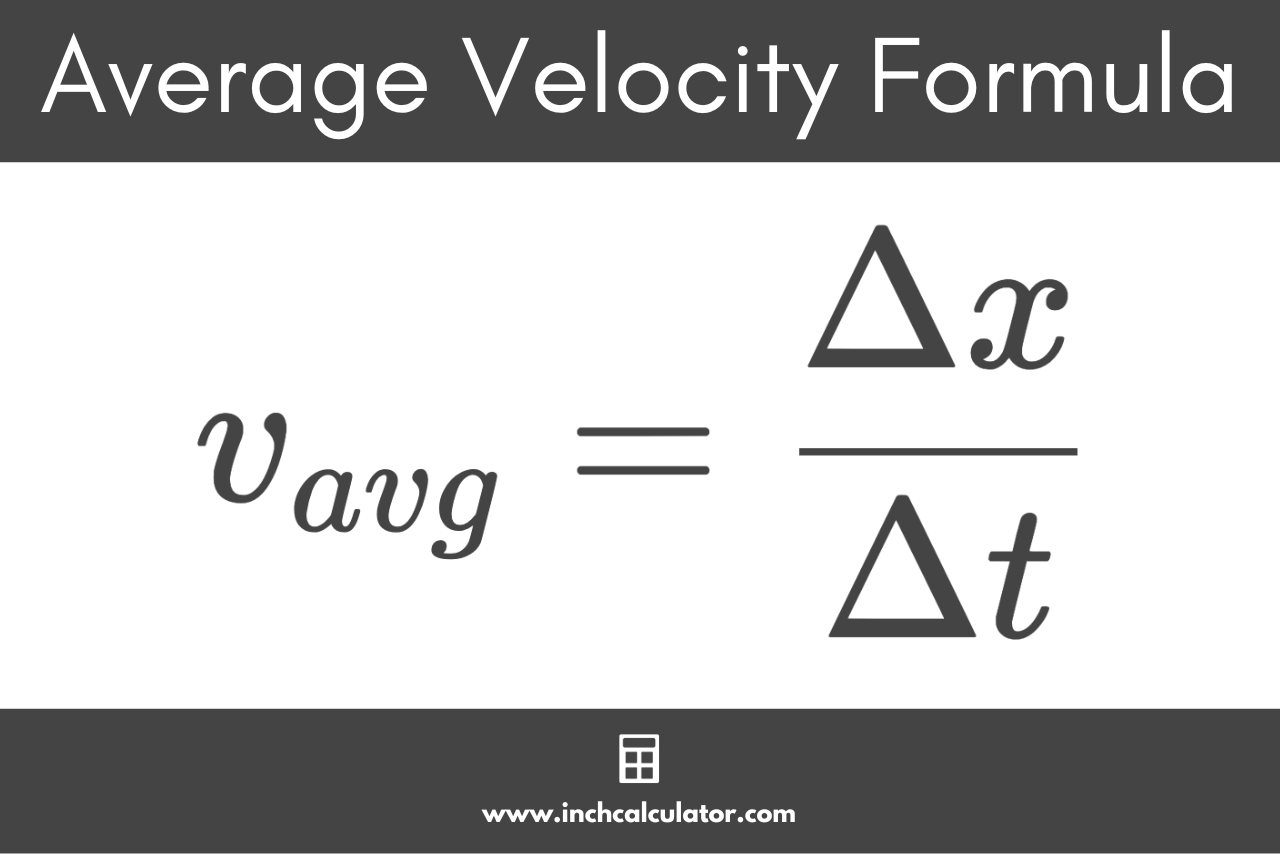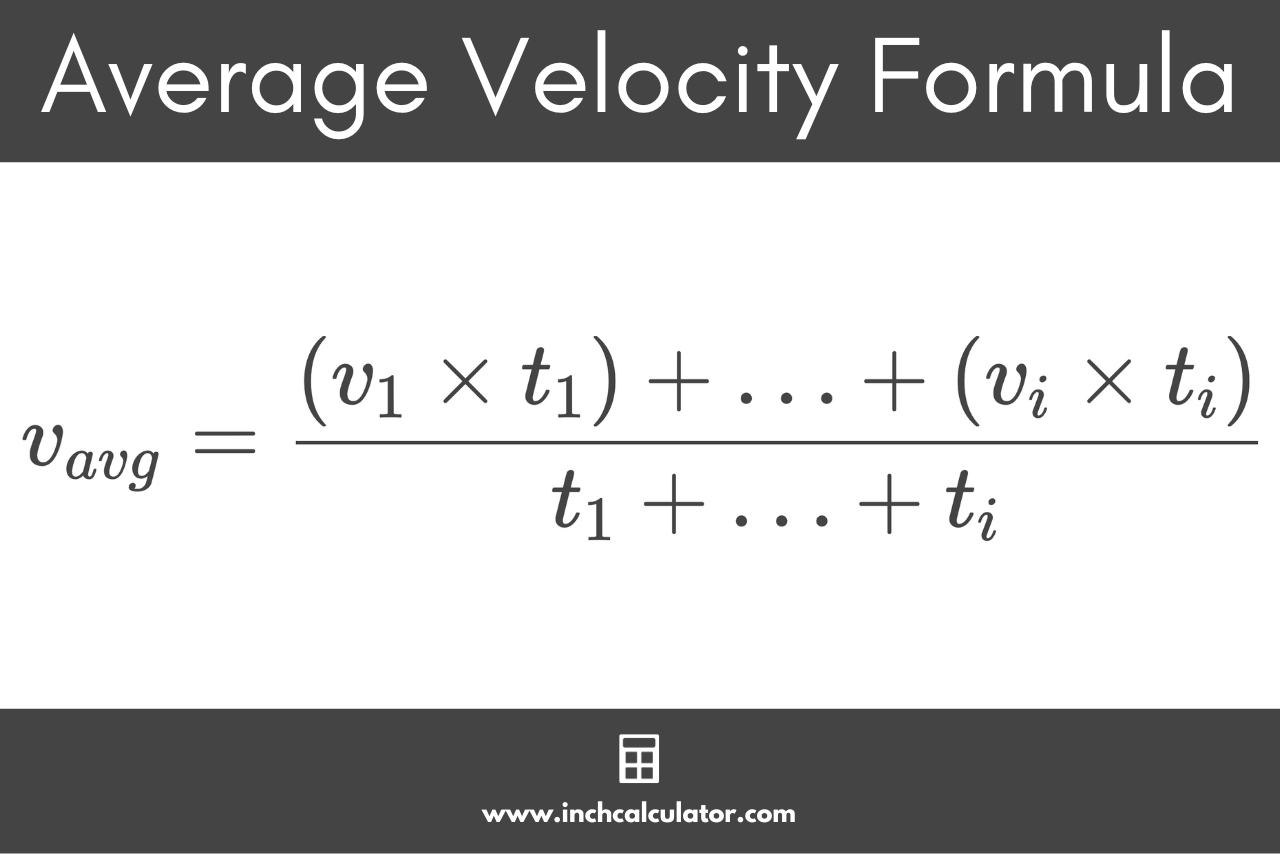# Average Velocity Calculator

Calculate the average velocity, final velocity, and initial velocity using the average velocity calculator below.

Calculate:

## Average Velocity:

-

### Average Velocity Formula

v_{avg}=\frac{v_{0}+v_{1}}{2}
Learn how we calculated this below

## How to Calculate Average Velocity

Velocity is a fundamental concept in physics that describes the rate that an object changes in position with respect to time. Essentially, velocity tells us how fast an object is moving and in which direction.

Velocity is a vector quantity, meaning it has both magnitude (speed) and direction.

The average velocity conveys the overall rate of change in the position of an object over a period of time, while instantaneous velocity conveys the rate of change at a specific moment.

There are several methods to calculate average velocity, depending on what information you have.

### Average Velocity Formula Using Displacement

The most common approach to calculating the average velocity is by using displacement. Displacement is the overall change in the position of an object, regardless of the direction or path taken.

If an object travels a certain displacement in a given time duration, then you can calculate the average velocity using the formula:

v_{avg}=\frac{\Delta x}{\Delta t}

The average velocity vavg is equal to the displacement Δx divided by the time duration Δt. The displacement is equal to the position of point one subtracted from the position of point two.

This is essentially the same method that’s used to calculate speed.You can also calculate the average velocity given multiple velocities using several formulas.

### Average Velocity Formula Using Two Velocities

If you know the two velocities, then you can calculate the average velocity using the following formula:

v_{avg}=\frac{v_{1}+v_{0}}{2}

The average velocity vavg is equal to the final velocity v1 plus the initial velocity v0, divided by 2.

### Average Velocity Formula Using More Than Two Velocities

If you know more than two velocities of an object, then you can calculate the average velocity using the average formula:

v_{avg}=\frac{(v_{1} \times t_{1}) + … + (v_{i} \times t_{i})}{t_{1} + … + t_{i}}

The average velocity vavg is equal to the sum of the products of the velocity vi and time duration ti for each time interval divided by the total time duration.